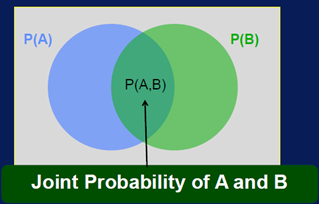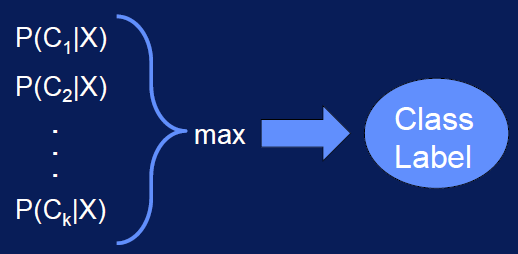# Naïve Bayes

## Definition:

• Naïve Bayes overview:
• Relationships between input features and class expressed as probabilities.
• Label for sample is class with highest probability given input.
• Naïve Bayes classifier:
• Classification using probability
• Bayes theorem: it makes estimating the probabilities easier.
• Feature independence assumption: For a given class, the value of one feature does not affect the value of any other feature.
• The naïve independence assumption and the use of Bayes theorem gives this classification model its name.
• Probability of event:
• Probability is measure of how likely an event is
• Probability of event ‘A’ occurring:• Joint probability:
• Probability of events A and B occurring together:• If the 2 events are independent: P(A, B) = P(A) * P(B)
• Conditional probability:
1. Probability of event A occurring, given that event B occurred.
2. Event A is conditioned on event B. P(A|B) = P(A,B)/P(B)

It provides the means to specify the probability of a class label, given the input values.

• Bayes’ theorem:
• Relationship between P(B|A) and P(A|B) can be expressed through Bayes’ theorem:• Classification with probabilities:

Given features X = {X1, X2,……,Xn}, predict class C. Do this by finding value of C that maximizes P(C|X)• Bayes theorem for classification:
• But estimating P(C|X) is difficult, we should use Bayes’ theorem to simplifies the problem:• So to get P(C|X), only need to find P(X|C) and P(C).
• Estimating P(C): To estimate P(C), calculate fraction of samples for class C in training data.
• Estimating P(X|C):
• Independence assumption: Features are independent of one another:

P (X1, X2,…Xn|C) = P(X1|C) * P(X2|C) * …….. * P(Xn|C)

• To estimate P(X|C), only need to estimate P(Xi|C) individually

• Naïve Bayes classification:
• Fast and simple: the probabilities that are needed can be calculated with a single scan of the data set and stored in a table.
• Scales well:
• Model building and testing of both task, it scales well.
• Due to the independent assumption:
1. The probability for each feature can be independently estimated.
2. Featured probability is can be calculated in very low.
3. The data set size does not have to grow exponentially with a number of features.
4. This avoid the many problems associated with the curse of dimensionality.
5. No need to a lot of data to build the model.
6. Number of parameters scales linearly with the number of features.